# DataWeave groupBy function: How to group items from Arrays, Strings, or Objects

We would like to thank MuleSoft Ambassador Joshua Erney for their contribution to this developer tutorial.

In this tutorial, you’ll learn some examples to group items from an Array, String, or Object using the `groupBy` function. If you come from a database background, you may know this function as `GROUP BY`. We’ll learn how to write the prefix and infix notations, as well as the use of an explicit lambda and the dollar-sign syntax.

You can try all of these examples with the DataWeave Playground. To learn more about it, check out this tutorial.

## Prerequisites

While not required to follow this tutorial, a good understanding of the basic DataWeave concepts would be preferred. You can check out these other tutorials if you feel a bit lost with some concepts:

## Syntax

The `groupBy` function is useful for grouping together items based on some value that you define. The function signature varies depending on the first parameter (whether it’s an Array, String, or Object).

Array

String

Object

`groupBy` does not return an Array or any of the given input values. It always returns an Object. When we define Object types, we can use two type parameters:

The first type parameter is the type of the keys (`K`), and the second type parameter is the type of the values (`T`).

Applying this to `groupBy`, we can see it returns an Object whose keys are the type of the values returned from the lambda (`R`), and the values are the type of the input data type (`Array<T>`, `String`, or `Object<(K), V>`).

No matter what type is used to create Object keys, they are always coerced to type Key. Even if the lambda returned a Number, the keys of the output Object would ultimately be of type Key.

## Prefix Notation

This function is not commonly used with the prefix notation because of its complexity. But here you can find an example that splits up odd and even numbers from an Array of Numbers.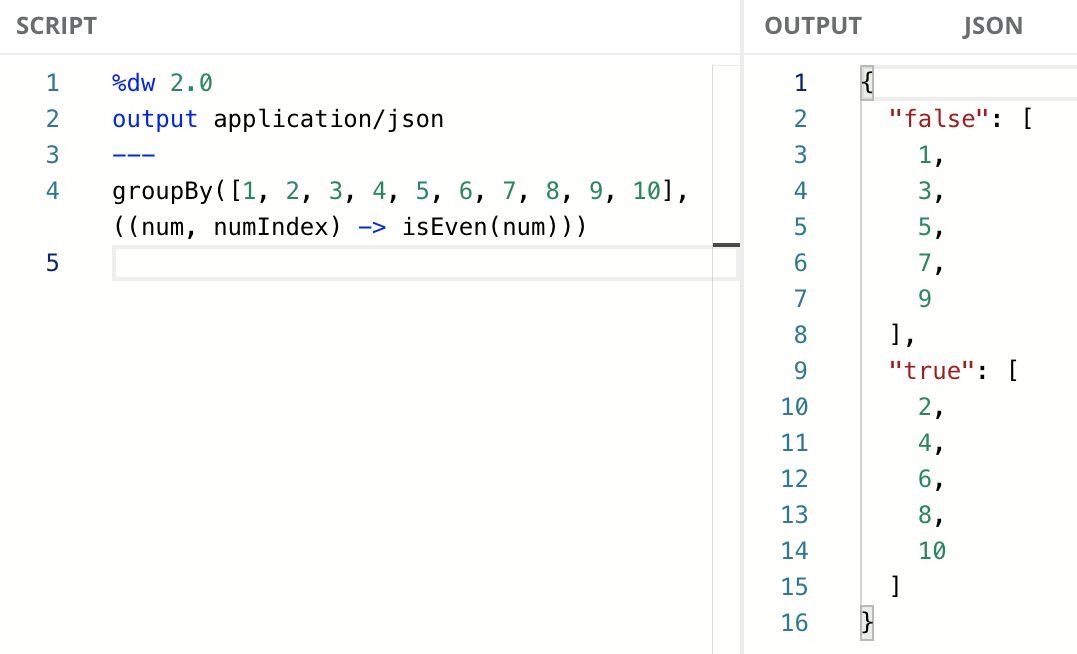If you’re not familiar with the `isEven` function, it returns `true` if the specified number is even, `false` if it’s not. You can learn more about it in the documentation.

## Infix Notation

This is the most used notation for this function because it makes it easier to read and understand.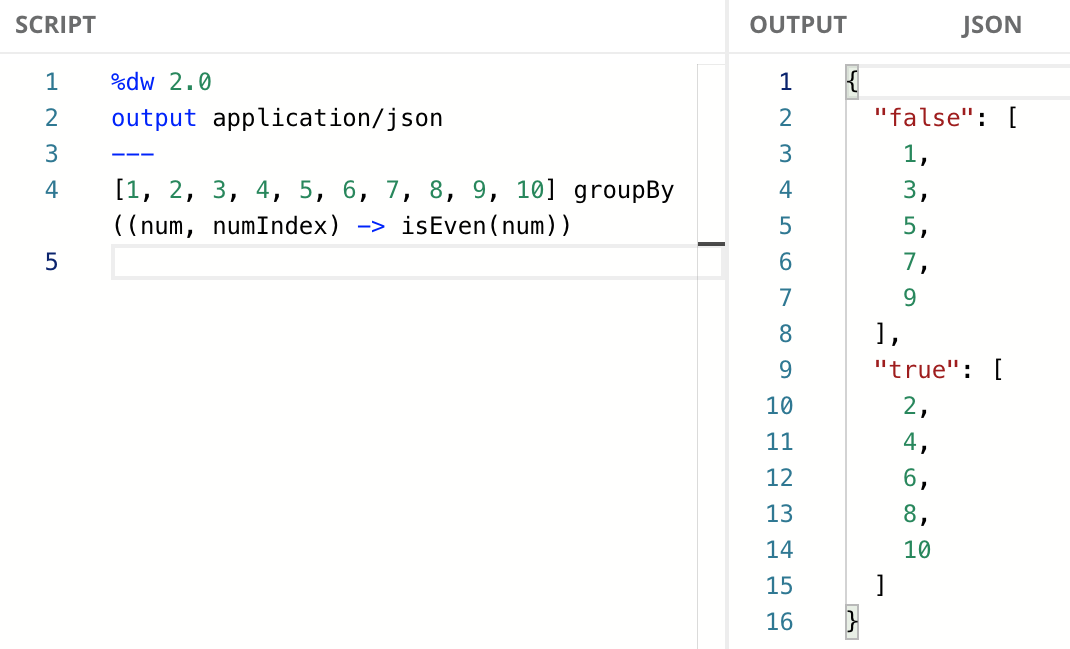When using the infix notation, you don’t have to include all the parameters in the lambda if you’re not using them. However, you do have to include the previous parameters (to the left) whether you’re using them or not. You can only remove the parameters to the right.

In this case, since `num` is the first parameter, we can also use the following code:

## Dollar-sign Syntax

You don’t always need to include the whole explicit lambda expression as the second argument. You can use the dollar-sign syntax to reference the two arguments that are passed to the lambda (i.e., `num` and `numIndex`). `\$` represents the value of the item (num), while `\$\$` represents the index (`numIndex`).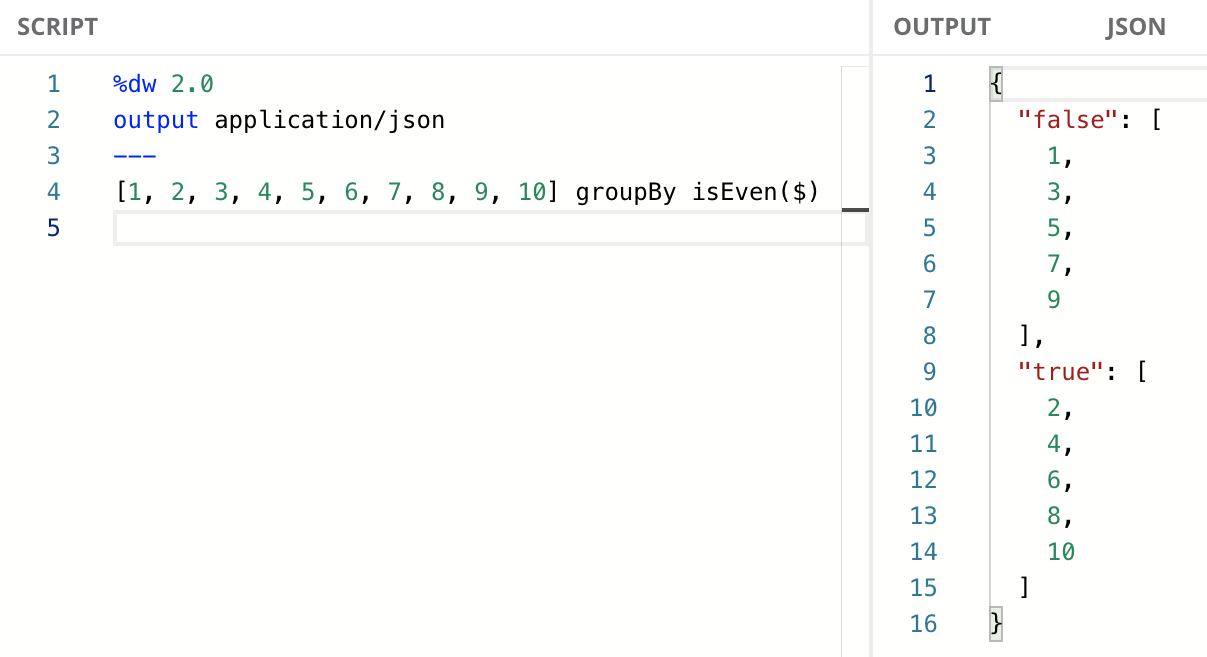## Group Items from an Array

The lambda passed to `groupBy` takes in an item from the input Array and the index of that item. It returns a value that is used to determine the group to which the item belongs. Items that return the same value belong to the same group. Here’s an example that groups calendar events based on the day of the week.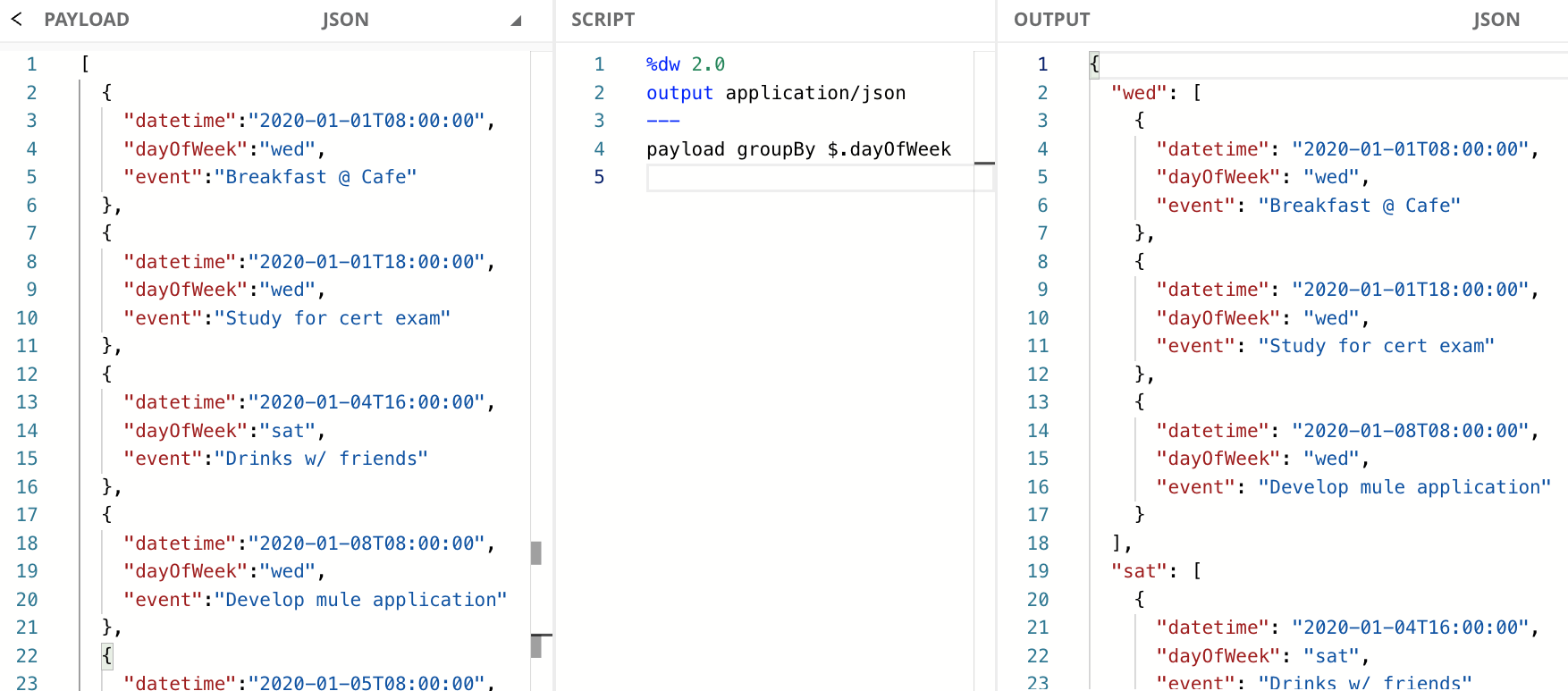In this example, we used the infix notation and the dollar-sign syntax. We chose to write it this way because the code looks cleaner. If you wanted to use the explicit lambda, while still using the infix notation, the same code would look like this:

## Group Characters from a String

The lambda passed to `groupBy` takes in a character from the input String and the index of that character. It returns a value that is used to determine the group to which the character belongs. Characters that return the same value belong to the same group. Here’s an example that groups letters based on whether they’re vocals or not.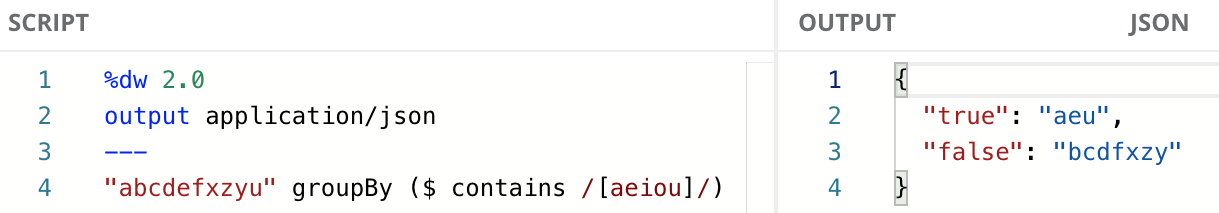Same as the previous example, we used the infix notation and the dollar-sign syntax. If you wanted to use explicit lambda and infix notation, the same code would look like this:

## Group Items from an Object

The lambda passed to `groupBy` takes in a value from the input Object and the key of that value. It returns a value that is used to determine the group to which the key/value pair belongs. Here’s an example that groups key/value pairs based on their value. The Key represents an employee (Josh, Pete, etc.) and the Value represents their manager (Jane, Bob, and Taylor).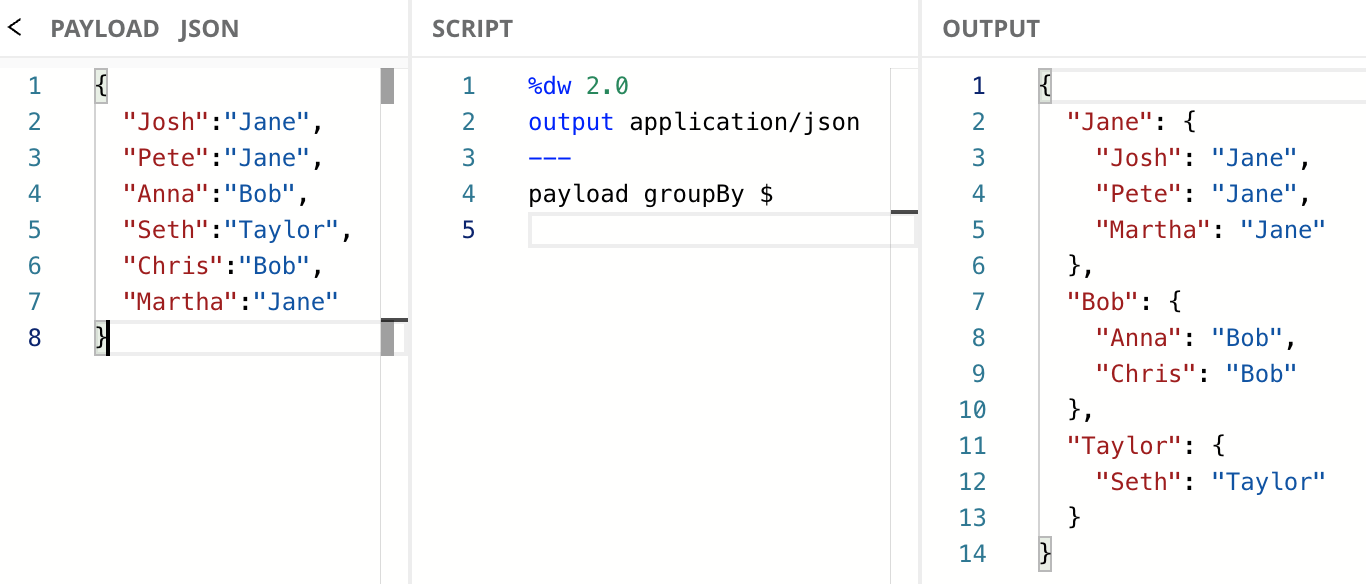Note that we used `\$` in the dollar-sign syntax because we are referencing the value from the key/value pair. If we wanted to group by the key instead, we would have to use `\$\$`. Note how on the previous examples (Array and String) `\$\$` refers to the index of the values. In this case, the function definition changes. If you wanted to use explicit lambda and infix notation with the previous example, it would look like this:

## Next Steps

In this tutorial, you learned some examples to group items from an Array, String, or Object using the `groupBy` function. You learned the function definitions for each of the input data and how to use them with the dollar-sign syntax.

Continue your development journey with the rest of the tutorials to become a master in DataWeave.